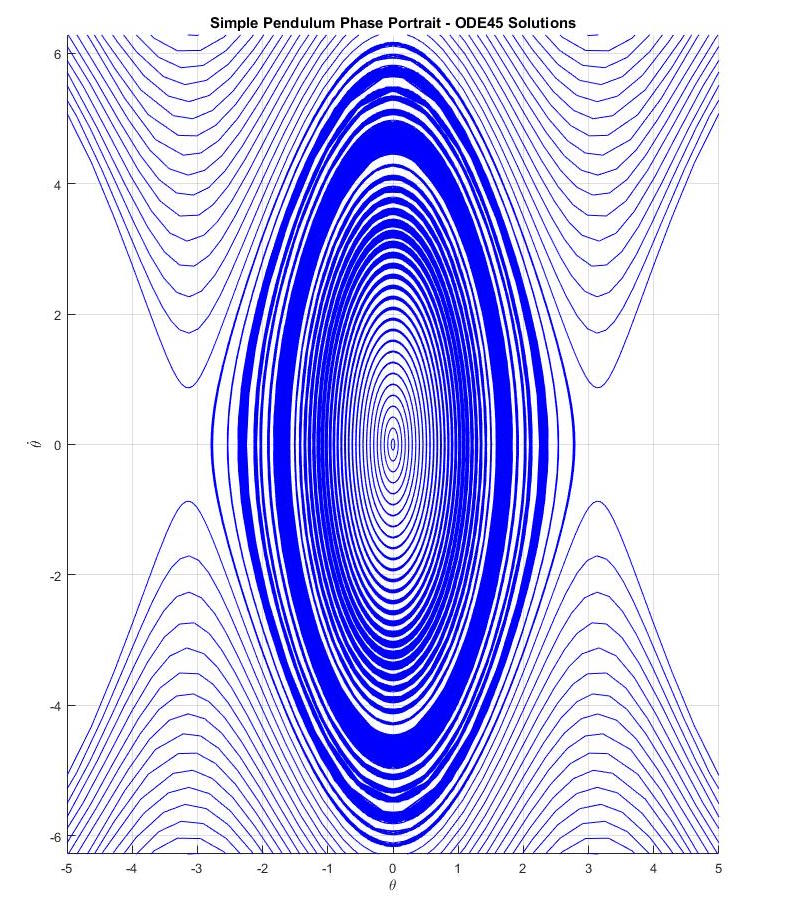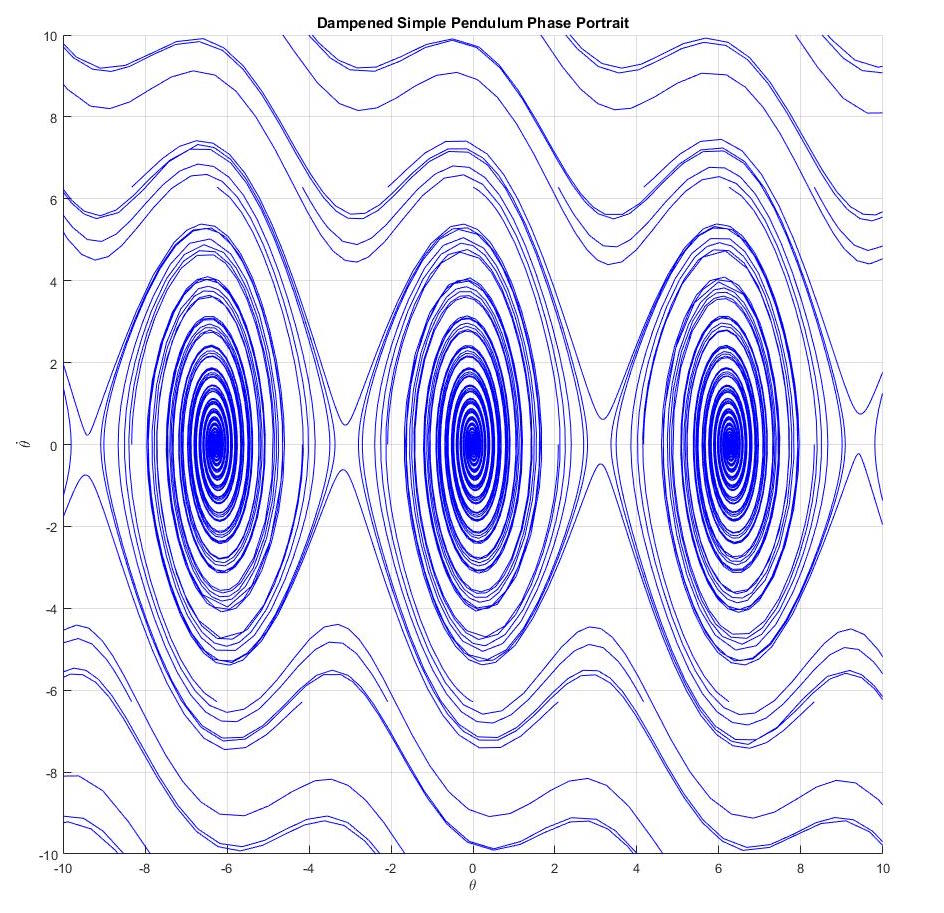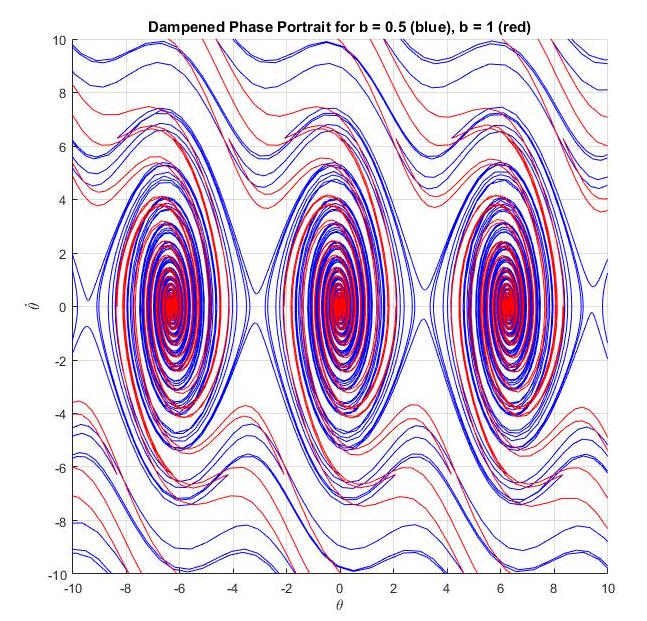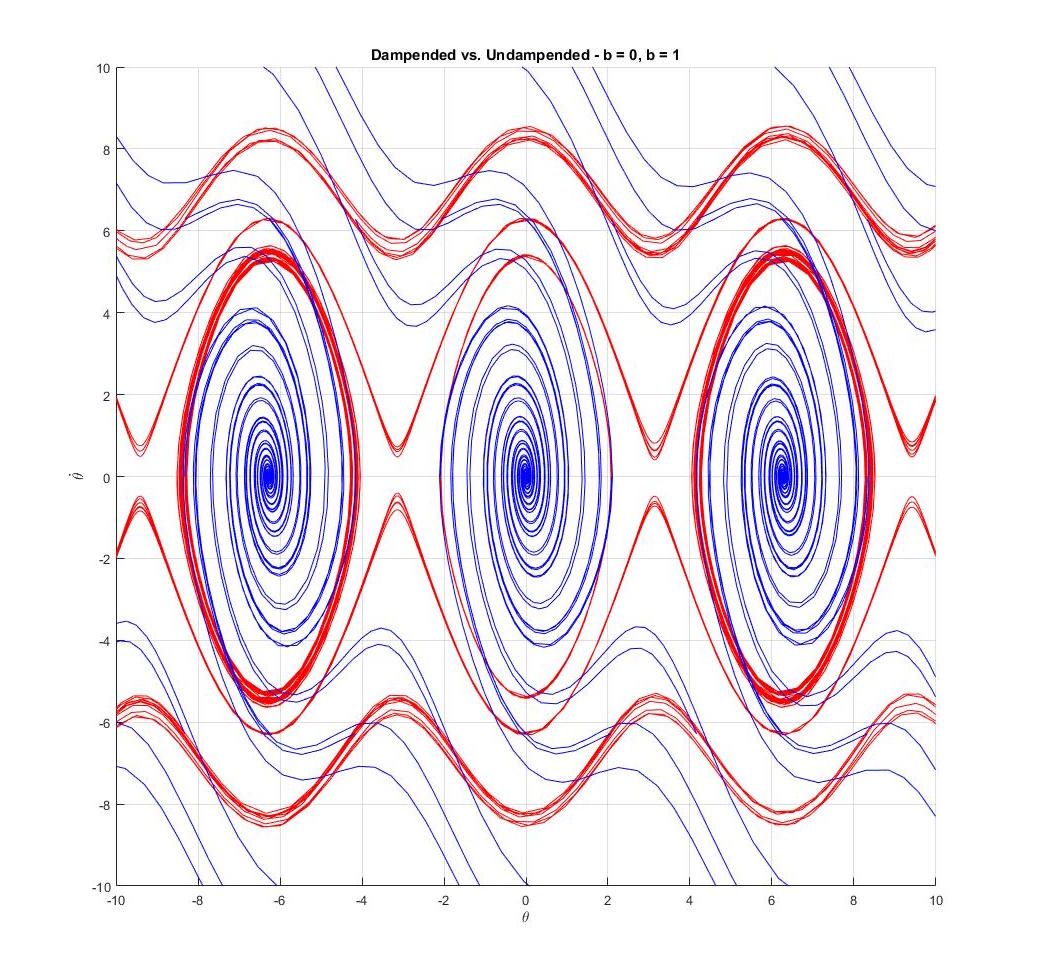# Simple Pendulum Phase Portraits

When investigating a given system's trajectories and dynamics it is often not intuitive how the system generally behaves. Even after solving the state space and finding the eigenvalues and eigenvectors that give information about the stability, it is still hard to visualize what is occurring for a given set of trajectories.

In the special case of second-order systems, a phase portrait is particularly useful. It plots the system trajectories as contours over a timespan along the X and Y axes which respectively represent the first and second order of the state space. In the case of nonlinear systems, phase portraits are particularly useful because they do no require any linearization or elimination of nonlinear terms. The phase portraits is able to perfectly capture all of the nonlinear trajectories and display them in a way that would be otherwise difficult.

The simple pendulum is a great example of a second-order nonlinear system that can be easily visualized by the phase portrait. The simple pendulum is often linearized using the small-angle approximation and converted into a linear form of zdot = A*z where A is a matrix of scalars. Instead, the phase portrait method leaves the simple pendulum state space in its nonlinear form as sin(θ).

It is worth noting that the inherent limitation of phase portraits is that they only applicable in second-order or lower systems. However, it cannot be asserted enough how effective phase portraits are at giving quick analysis for even highly-nonlinear systems.

The code below provide phase portraits for various dampened and undampened simple pendulum scenarios. Notice how the dampened trajectories converge to a point while the undampened ones form concentric contours, formally known as limit cycles. Also, notice how for the larger dampening coefficient, the trajectories converge to a point much quicker, with fewer rotations around. This reflects our intution that a pendulum with more friction (a larger dampening coefficient) will come to rest faster. Similarly, the limit cylces formed in the undampened figures show that without any friction, the pendulum will continue to swing around the equilibrium point forever. It is clear why phase portraits are a powerful tool for analyzing nonlinear systems, read more about them here.


% Script for Plotting the Phase Portrait of a Nonlinear Pendulum

% init system and states
g = 9.81;
L = 1;
ss = @(t,THETA) [THETA(2); -g./L*sin(THETA(1))];
THETA1 = linspace(-2*pi,2*pi,50);
THETA2 = linspace(-2*pi,2*pi,25);

% Generate Meshgrid for numerically solving
[x,y] = meshgrid(THETA1,THETA2);
size(x)
size(y)

% Establish U and V vectors
u = zeros(size(x));
v = zeros(size(y));

% Init Loop over Vector field to compute Derivatives
t = 0;
for i = 1:numel(x)
Theta_d = ss(t,[x(i); y(i)]);
u(i) = Theta_d(1);
v(i) = Theta_d(2);
Mag = sqrt(u(i)^2+v(i)^2);
%u(i) = u(i)/Mag;
%v(i) = v(i)/Mag;
end

%Plot our vector field
quiver(x,y,u,v,'r'); figure(gcf)
xlabel('${\theta}$','interpreter','latex')
ylabel('$\dot{\theta}$','interpreter','latex')
axis tight equal;

hold on

% Plotting Solutions for Comparison
for THETA_sol = linspace(-4*pi,4*pi,150)
[ts,ys] = ode45(ss,[0,50],[0;THETA_sol]);
plot(ys(:,1),ys(:,2),'b')
plot(-ys(:,1),ys(:,2),'b')
%plot(ys(1,1),ys(1,2),'bo') %start of contour
%plot(ys(end,1),ys(end,2),'ks') %end of contour
end

axis([-5 5 -2*pi 2*pi])
grid on

hold off


% Script for Plotting the Phase Portrait of a Dampened Nonlinear Pendulum
% Change the state space to include "b" dampening coefficient

% init system and states
g = 9.81;
L = 1;
b = 1;
ss = @(t,THETA) [THETA(2); -b.*THETA(2)-g./L*sin(THETA(1))];
THETA1 = linspace(-2*pi,2*pi,50);
THETA2 = linspace(-2*pi,2*pi,25);